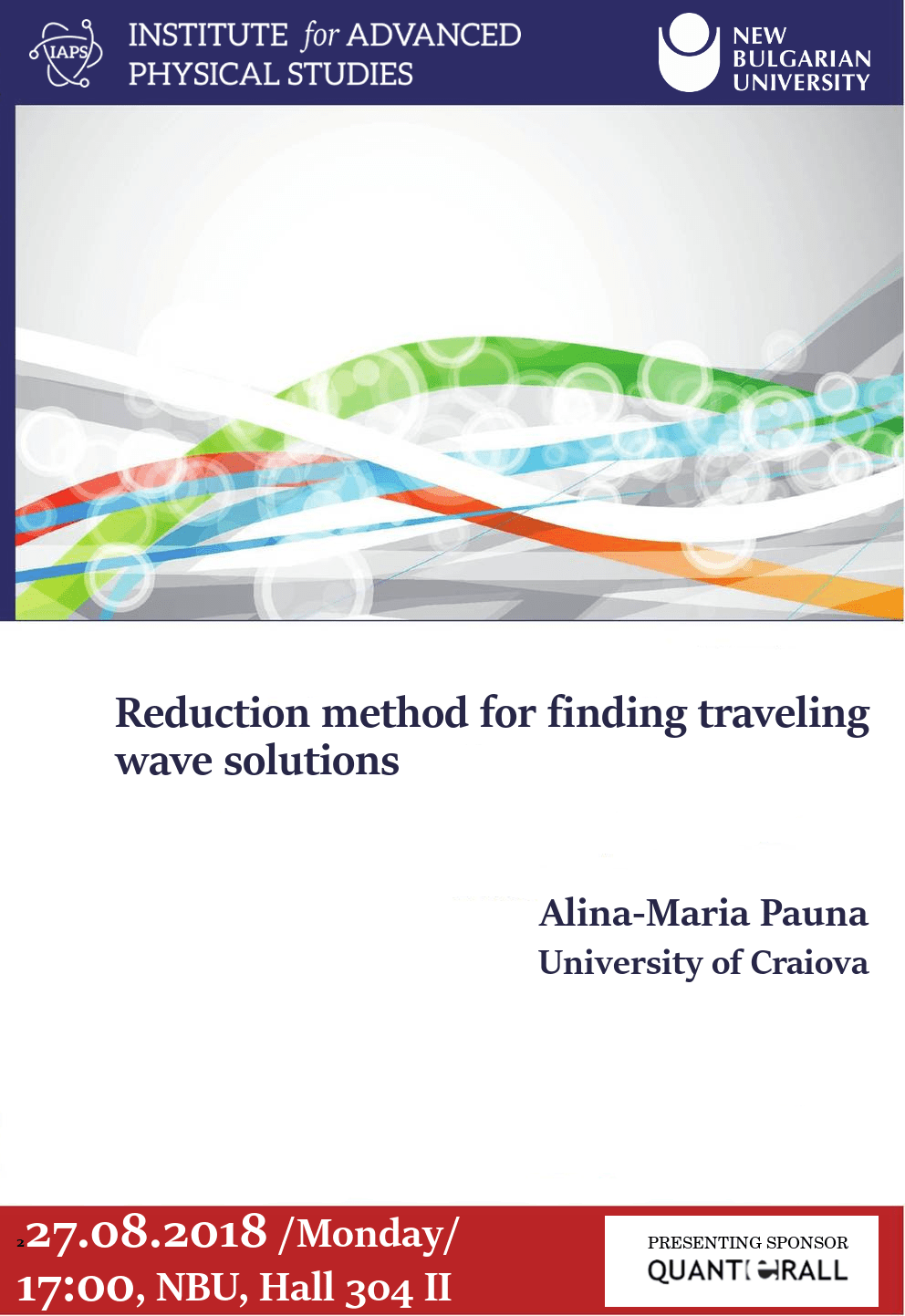Select PageLECTURER: Alina-Maria Pauna, University of Craiova

TIME: 27.08.2018 /MONDAY/ 17:00

VENUE: NEW BULGARIAN UNIVERSITY, HALL 304, BUILDING 2

We consider nonlinear partial differential equations which are encountered in different fields of mathematics, physics, chemistry and biology. A reduction method of the initial equations based on the attached flow-type equations is proposed. First, we reduce the partial differential equation to an ordinary differential equation (ODE) using the traveling wave transformation $\xi=x+\lambda t$, $u(x,t) = U(\xi)$. Next we find special solutions of the resulting ODE by requesting that $U’=V(U)$ where $V(U)$ may be a polynomial of $U$. The method is applied to the Benjamin-Bona-Mahony eq., generalized Boussinesq eq., Tzitzeica eq. and Whitman-Brower-Kaup system. Their traveling wave solutions are constructed. The advantage of the current method is that it is simple, direct, elementary and concise.